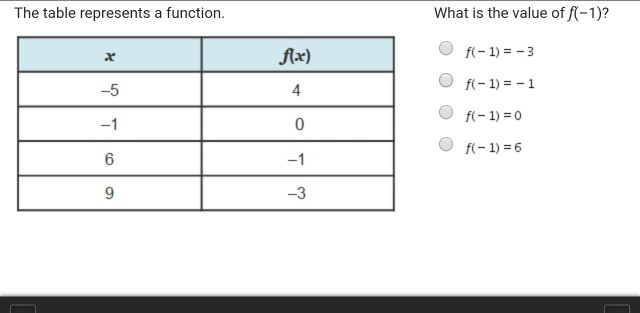# The table represents a function what is the value of f(-1)The table represents a function What is the value of f(-1)? fax) 4 0 -5 f(-1) =0 f(-1) = 6 9 -3

As per the table given , depicting values of f(x) when x =
-5,-1,6 and 9, the value of f(x) when x = -1 is 0. Thus, f(-1)= 0.
The 3rd option is the correct answer.